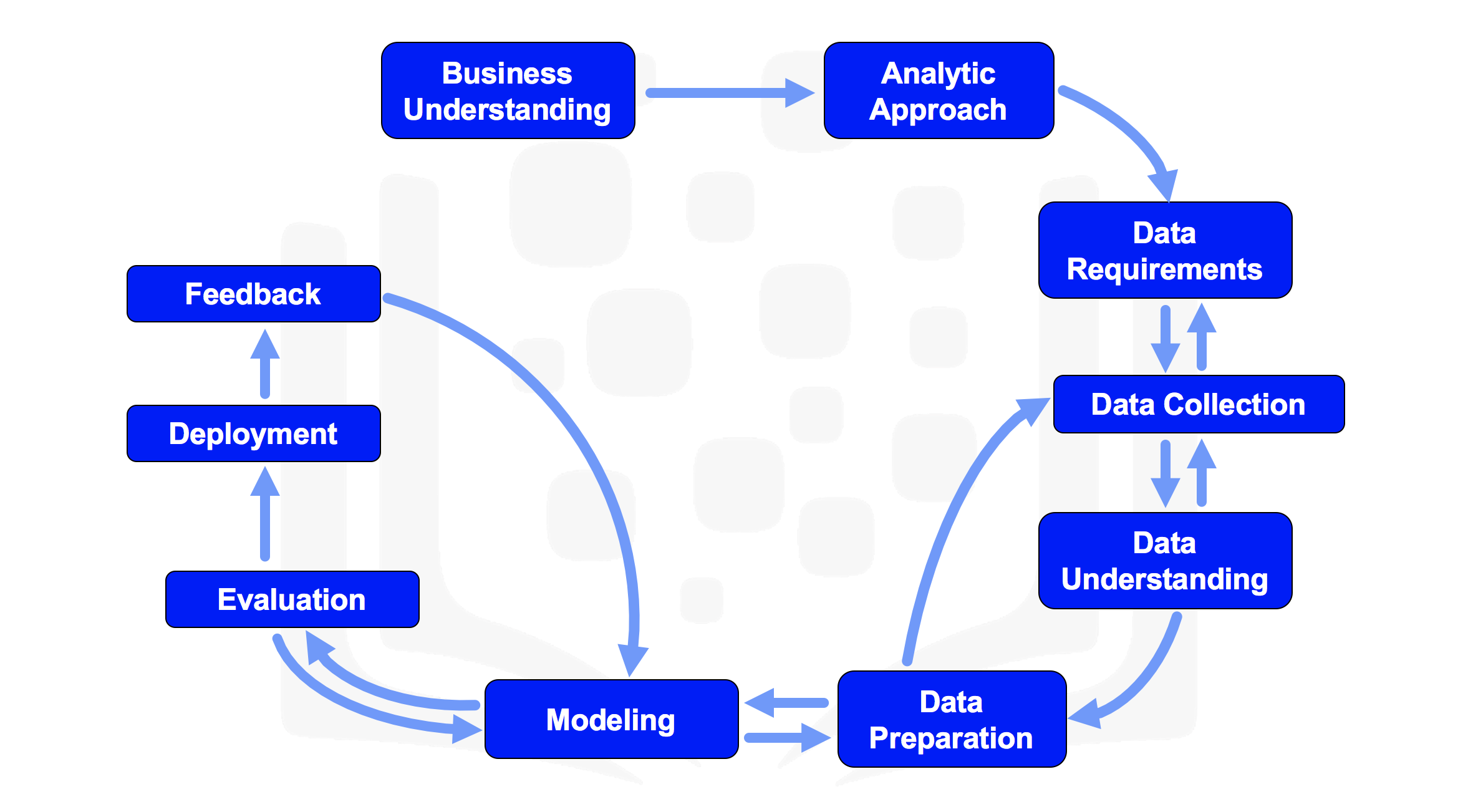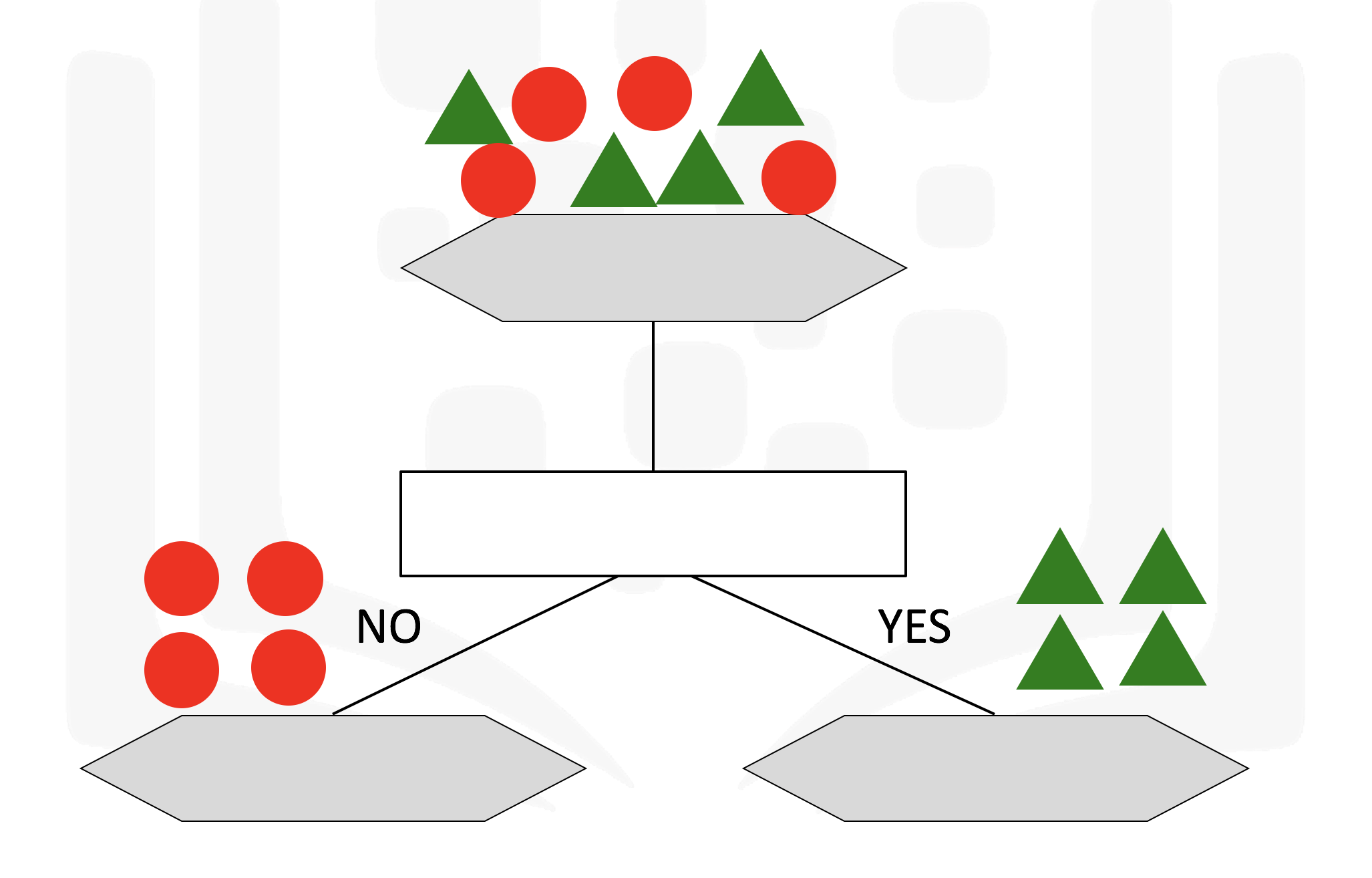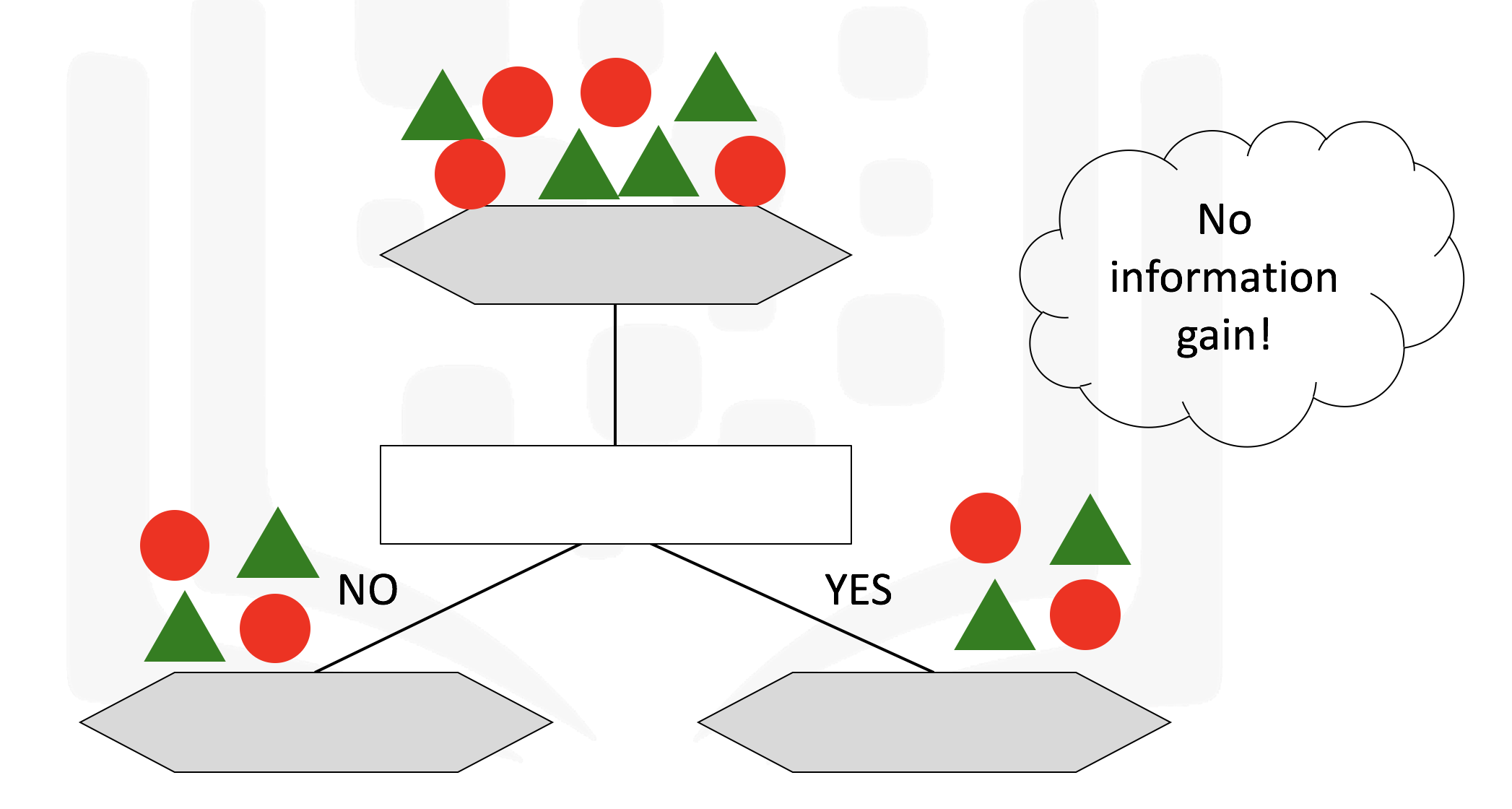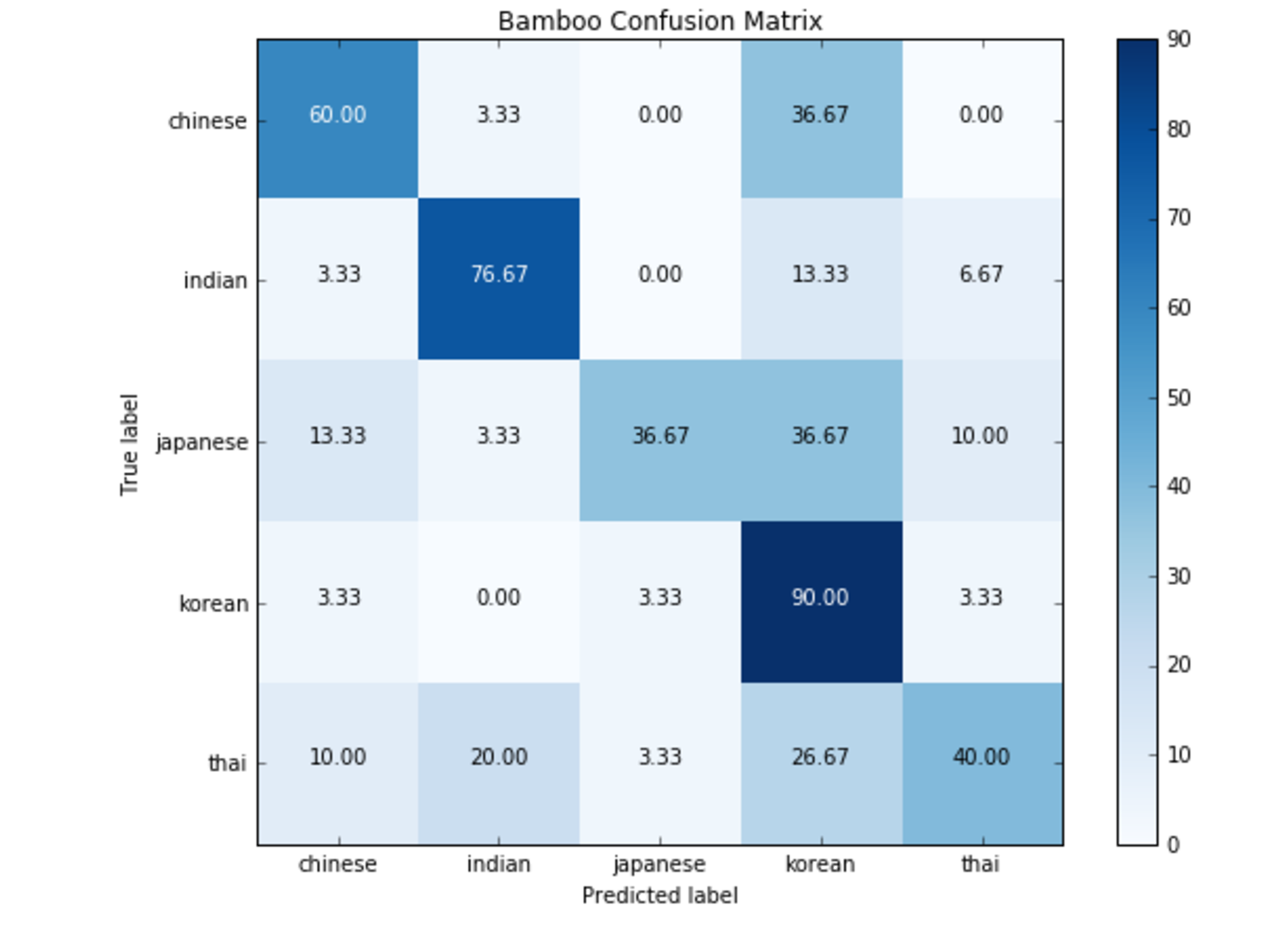# Data Science Methodology

Created:

Updated: 03 September 2023

Based on this Cognitive Class Course

# The Methodology

The data science methodology described here is as outlined by John Rollins of IBM

The Methodology can be seen in the following steps# The Questions

The Data Science methodology aims to answer ten main questions

## From Problem to Approach

1. What is the problem you are trying to solve?
2. How can you use data to answer the question?

## Working with the Data

1. What data do you need to answer the question?>
2. Where is the data coming from and how will you get it?
3. Is the data that you collected representative of the problem to be solved?
4. What additional work is required to manipulate and work with the data

1. In what way can the data be visualized to get to the answer that is required?
2. Does the model used really answer the initial question or does it need to be adjusted?
3. Can you put the model into practice?
4. Can you get constructive feedback into answering the question?

# Problem and Approach

What is the problem you are trying to solve?

The firs step in the methodology involves seeking any needed clarification in order to identify what the problem we are trying to solve is as this drives the data we use and the analytical approach that we will go about applying

It is important to seek clarification early on otherwise we can waste time and resources moving in the wrong direction

In order to understand a question, it is important to understand the goal of the person asking the question

Based on this we will break down objectives and prioritize them

## Analytic Approach

How can you use data to answer the question?

The second step in the methodology is electing the correct approach involves the specific problem being addressed, this points to the purpose of business understanding and helps us to identify what methods we should use in order to address the problem

### Approach to be Used

When we have a strong understanding of the problem, wwe can pick an analytical approach to be used

• Descriptive
• Current Status
• Diagnostic (Statistical Analysis)
• What happened?
• Why is this happening?
• Predictive (Forecasting)
• What if these trends continue?
• What will happen next?
• Prescriptive
• How do we solve it?

### Question Types

We have a few different types of questions that can direct our modelling

• Question is to determine probabilities of an action
• Predictive Model
• Question is to show relationships
• Descriptive Model
• Question requires a binary answer
• Classification Model

### Machine Learning

Machine learning allows us to identify relationships and trends that cannot otherwise be established

### Decision Trees

Decision trees are a machine learning algorithm that allow us to classify nodes while also giving us some information as to how the information is classified

It makes use of a tree structure with recursive partitioning to classify data, predictiveness is based on decrease in entropy - gain in information or impurity

A decision tree for classifying data can result in leaf nodes of varying purity, as seen below which will provide us with different ammounts of informationSome of the characteristics of decision trees are summarized below

ProsCons
Easy to interpretEasy to over or underfit the model
Can handle numeric or categorical featuresCannot model feature interaction
Can handle missing dataLarge trees can be difficult to interpret
Uses only the most important features
Can be used on very large or small datasets

## Labs

The Lab notebooks have been added in the labs folder, and are released under the MIT License

The Lab for this section is 1-From-Problem-to-Approach.ipynb

# Requirements and Collection

## Data Requirements

What data do you need to answer the question?

We need to understand what data is required, how to collect it, and how to transform it to address the problem at hand

It is necessary to identify the data requirements for the initial data collection

We typically make use of the following steps

1. Define and select the set of data needed
2. Content format and representation of data was defined
3. It is important to look ahead when transforming our data to a form that would be most suitable for us

## Data Collection

Where is the data coming from and how will you get it?

After the initial data collection has been performed, we look at the data and verify that we have all the data that we need, and the data requirements are revisited in order to define what has not been met or needs to be changed

We then make use of descriptive statistics and visuals in order to define the quality and other aspects of the data and then identify how we can fill in these gaps

Collecting data requires that we know the data source and where to find the required data

## Labs

The lab documents for this section are in both Python and R, and can be found in the labs folder as 2-Requirements-to-Collection-py.ipynb and 2-Requirements-to-Collection-R.ipynb

These labs will simply read in a dataset from a remote source as a CSV and display it

### Python

In Python we will use Pandas to read data as DataFrames

We can use Pandas to read data into the data frame

import pandas as pd # download library to read data into dataframe
pd.set_option('display.max_columns', None)

print("Data read into dataframe!") # takes about 30 seconds

Thereafter we can view the dataframe by looking at the first few rows, as well as the dimensions with

recipes.head()
recipes.shape

### R

We do the same aas the above in R as follows

# click here and press Shift + Enter
destfile = "/resources/data/recipes.csv", quiet = TRUE)

print("Done!") # takes about 30 seconds

Thereafter we can read this into a variable with

recipes <- read.csv("/resources/data/recipes.csv") # takes 10 sec

We can then see the first few rows of data as well as the dimensions with

head(recipes)
nrow(recipes)
ncol(recipes)

# Understanding and Preparation

## Data Understanding

Is the data that you collected representative of the problem to be solved?

We make use of descriptive statistics to understand the data

We run statistical analyses to learn about the data with means such as such as

• Univariate
• Pairwise
• Histogram
• Mean
• Medium
• Min
• Max
• etc.

We also make use of these to understand data quality and values such as Missing values and Invalid or Misleading values

## Data Preparation

What additional work is required to manipulate and work with the data

Data preparation is similar to cleansing data by removing unwanted elements and imperfections, this can take between 70% and 90% of the project time

Transforming data in this phase is the process of turining data into something that would be easier to work with

Some examples of what we need to look out for are

• Invalid values
• Missing data
• Duplicates
• Formatting

Another part of data preparation is feature engineering which is when we use domain knowledge to create features for our predictive models

The data preparation will support the remainder of the project

## Labs

The lab documents for this section are in both Python and R, and can be found in the labs folder as 3-Understanding-to-Preparation-py.ipynb and 3-Understanding-to-Preparation-R.ipynb

These labs will continue to analyze the data that was imported from the previous lab

### Python

First, we check if the ingredients exist in our dataframe

ingredients = list(recipes.columns.values)

print([match.group(0) for ingredient in ingredients for match in [(re.compile(".*(rice).*")).search(ingredient)] if match])
print([match.group(0) for ingredient in ingredients for match in [(re.compile(".*(wasabi).*")).search(ingredient)] if match])
print([match.group(0) for ingredient in ingredients for match in [(re.compile(".*(soy).*")).search(ingredient)] if match])

Thereafter we can look at our data in order to see if there are any changes that need to be made

recipes["country"].value_counts() # frequency table

# American                   40150
# Mexico                      1754
# Italian                     1715
# Italy                       1461
# Asian                       1176
# French                       996
# east_asian                   951
# korean                       767
# Mexican                      622
# western                      450
# Southern_SoulFood            346
# India                        324
# Jewish                       320
# Spanish_Portuguese           291

From here the following can be seen

1. Cuisine is labelled as country
2. Cuisine names are not consistent, uppercase, lowercase, etc.
3. Some cuisines are duplicates of the country name
4. Some cuisines have very few recipes

We can take a few steps to solve these problems

First we fix the Country title to be Cuisine

column_names = recipes.columns.values
column_names = "cuisine"
recipes.columns = column_names

recipes

Then we can make all the names lowercase

recipes["cuisine"] = recipes["cuisine"].str.lower()

Next we correct the mislablled cuisine names

recipes.loc[recipes["cuisine"] == "austria", "cuisine"] = "austrian"
recipes.loc[recipes["cuisine"] == "belgium", "cuisine"] = "belgian"
recipes.loc[recipes["cuisine"] == "china", "cuisine"] = "chinese"
recipes.loc[recipes["cuisine"] == "netherlands", "cuisine"] = "dutch"
recipes.loc[recipes["cuisine"] == "france", "cuisine"] = "french"
recipes.loc[recipes["cuisine"] == "germany", "cuisine"] = "german"
recipes.loc[recipes["cuisine"] == "india", "cuisine"] = "indian"
recipes.loc[recipes["cuisine"] == "indonesia", "cuisine"] = "indonesian"
recipes.loc[recipes["cuisine"] == "iran", "cuisine"] = "iranian"
recipes.loc[recipes["cuisine"] == "italy", "cuisine"] = "italian"
recipes.loc[recipes["cuisine"] == "japan", "cuisine"] = "japanese"
recipes.loc[recipes["cuisine"] == "israel", "cuisine"] = "jewish"
recipes.loc[recipes["cuisine"] == "korea", "cuisine"] = "korean"
recipes.loc[recipes["cuisine"] == "lebanon", "cuisine"] = "lebanese"
recipes.loc[recipes["cuisine"] == "malaysia", "cuisine"] = "malaysian"
recipes.loc[recipes["cuisine"] == "mexico", "cuisine"] = "mexican"
recipes.loc[recipes["cuisine"] == "pakistan", "cuisine"] = "pakistani"
recipes.loc[recipes["cuisine"] == "philippines", "cuisine"] = "philippine"
recipes.loc[recipes["cuisine"] == "scandinavia", "cuisine"] = "scandinavian"
recipes.loc[recipes["cuisine"] == "spain", "cuisine"] = "spanish_portuguese"
recipes.loc[recipes["cuisine"] == "portugal", "cuisine"] = "spanish_portuguese"
recipes.loc[recipes["cuisine"] == "switzerland", "cuisine"] = "swiss"
recipes.loc[recipes["cuisine"] == "thailand", "cuisine"] = "thai"
recipes.loc[recipes["cuisine"] == "turkey", "cuisine"] = "turkish"
recipes.loc[recipes["cuisine"] == "vietnam", "cuisine"] = "vietnamese"
recipes.loc[recipes["cuisine"] == "uk-and-ireland", "cuisine"] = "uk-and-irish"
recipes.loc[recipes["cuisine"] == "irish", "cuisine"] = "uk-and-irish"

recipes

After that we can remove the cuisines with less than 50 recipes

# get list of cuisines to keep
recipes_counts = recipes["cuisine"].value_counts()
cuisines_indices = recipes_counts > 50

cuisines_to_keep = list(np.array(recipes_counts.index.values)[np.array(cuisines_indices)])

And then view the number of rows we kepy/removed

rows_before = recipes.shape # number of rows of original dataframe
print("Number of rows of original dataframe is {}.".format(rows_before))

recipes = recipes.loc[recipes['cuisine'].isin(cuisines_to_keep)]

rows_after = recipes.shape # number of rows of processed dataframe
print("Number of rows of processed dataframe is {}.".format(rows_after))

print("{} rows removed!".format(rows_before - rows_after))

Next we can convert the yes/no fields to be binary

recipes = recipes.replace(to_replace="Yes", value=1)
recipes = recipes.replace(to_replace="No", value=0)

And lastly view our data with

recipes.head()

Next we can Look for recipes that contain rice and soy and wasabi and seaweed

check_recipes = recipes.loc[
(recipes["rice"] == 1) &
(recipes["soy_sauce"] == 1) &
(recipes["wasabi"] == 1) &
(recipes["seaweed"] == 1)
]

check_recipes

Based on this we can see that not all recipes with those ingredients are Japanese

Now we can look at the frequency of different ingredients in these recipes

# sum each column
ing = recipes.iloc[:, 1:].sum(axis=0)
# define each column as a pandas series
ingredient = pd.Series(ing.index.values, index = np.arange(len(ing)))
count = pd.Series(list(ing), index = np.arange(len(ing)))

# create the dataframe
ing_df = pd.DataFrame(dict(ingredient = ingredient, count = count))
ing_df = ing_df[["ingredient", "count"]]
print(ing_df.to_string())

We can then sort the dataframe of ingredients in descending order

# define each column as a pandas series
ingredient = pd.Series(ing.index.values, index = np.arange(len(ing)))
count = pd.Series(list(ing), index = np.arange(len(ing)))

# create the dataframe
ing_df = pd.DataFrame(dict(ingredient = ingredient, count = count))
ing_df = ing_df[["ingredient", "count"]]
print(ing_df.to_string())

From this we can see that the most common ingredients are Egg, Wheat, and Butter. However we have a lot more American recipes than the others, indicating that our data is skewed towards American ingredients

We can now create a profile for each cuisine in order to see a more representative recipe distribution with

cuisines = recipes.groupby("cuisine").mean()
cuisines.head()

We can then print out the top 4 ingredients of every couisine with the following

num_ingredients = 4 # define number of top ingredients to print

# define a function that prints the top ingredients for each cuisine
def print_top_ingredients(row):
print(row.name.upper())
row_sorted = row.sort_values(ascending=False)*100
top_ingredients = list(row_sorted.index.values)[0:num_ingredients]
row_sorted = list(row_sorted)[0:num_ingredients]

for ind, ingredient in enumerate(top_ingredients):
print("%s (%d%%)" % (ingredient, row_sorted[ind]), end=' ')
print("\n")

# apply function to cuisines dataframe
create_cuisines_profiles = cuisines.apply(print_top_ingredients, axis=1)

# AFRICAN
# onion (53%) olive_oil (52%) garlic (49%) cumin (42%)

# AMERICAN
# butter (41%) egg (40%) wheat (39%) onion (29%)

# ASIAN
# soy_sauce (49%) ginger (48%) garlic (47%) rice (41%)

# CAJUN_CREOLE
# onion (69%) cayenne (56%) garlic (48%) butter (36%)

# wheat (39%) butter (38%) egg (35%) onion (34%)

### R

First, we check if the ingredients exist in our dataframe

grep("rice", names(recipes), value = TRUE) # yes as rice
grep("wasabi", names(recipes), value = TRUE) # yes
grep("soy", names(recipes), value = TRUE) # yes as soy_sauce

Thereafter we can look at our data in order to see if there are any changes that need to be made

base::table(recipes$country) # frequency table # American 40150 # Mexico 1754 # Italian 1715 # Italy 1461 # Asian 1176 # French 996 # east_asian 951 # Canada 774 # korean 767 # Mexican 622 # western 450 # Southern_SoulFood 346 # India 324 # Jewish 320 # Spanish_Portuguese 291 From here the following can be seen 1. Cuisine is labelled as country 2. Cuisine names are not consistent, uppercase, lowercase, etc. 3. Some cuisines are duplicates of the country name 4. Some cuisines have very few recipes We can take a few steps to solve these problems First we fix the Country title to be Cuisine colnames(recipes) = "cuisine" Then we can make all the names lowercase recipes$cuisine <- tolower(as.character(recipes$cuisine)) recipes Next we correct the mislablled cuisine names recipes$cuisine[recipes$cuisine == "austria"] <- "austrian" recipes$cuisine[recipes$cuisine == "belgium"] <- "belgian" recipes$cuisine[recipes$cuisine == "china"] <- "chinese" recipes$cuisine[recipes$cuisine == "canada"] <- "canadian" recipes$cuisine[recipes$cuisine == "netherlands"] <- "dutch" recipes$cuisine[recipes$cuisine == "france"] <- "french" recipes$cuisine[recipes$cuisine == "germany"] <- "german" recipes$cuisine[recipes$cuisine == "india"] <- "indian" recipes$cuisine[recipes$cuisine == "indonesia"] <- "indonesian" recipes$cuisine[recipes$cuisine == "iran"] <- "iranian" recipes$cuisine[recipes$cuisine == "israel"] <- "jewish" recipes$cuisine[recipes$cuisine == "italy"] <- "italian" recipes$cuisine[recipes$cuisine == "japan"] <- "japanese" recipes$cuisine[recipes$cuisine == "korea"] <- "korean" recipes$cuisine[recipes$cuisine == "lebanon"] <- "lebanese" recipes$cuisine[recipes$cuisine == "malaysia"] <- "malaysian" recipes$cuisine[recipes$cuisine == "mexico"] <- "mexican" recipes$cuisine[recipes$cuisine == "pakistan"] <- "pakistani" recipes$cuisine[recipes$cuisine == "philippines"] <- "philippine" recipes$cuisine[recipes$cuisine == "scandinavia"] <- "scandinavian" recipes$cuisine[recipes$cuisine == "spain"] <- "spanish_portuguese" recipes$cuisine[recipes$cuisine == "portugal"] <- "spanish_portuguese" recipes$cuisine[recipes$cuisine == "switzerland"] <- "swiss" recipes$cuisine[recipes$cuisine == "thailand"] <- "thai" recipes$cuisine[recipes$cuisine == "turkey"] <- "turkish" recipes$cuisine[recipes$cuisine == "irish"] <- "uk-and-irish" recipes$cuisine[recipes$cuisine == "uk-and-ireland"] <- "uk-and-irish" recipes$cuisine[recipes$cuisine == "vietnam"] <- "vietnamese" recipes After that we can remove the cuisines with less than 50 recipes # sort the table of cuisines by descending order # get cuisines with >= 50 recipes filter_list <- names(t[t >= 50]) filter_list And then view the number of rows we kept/removed # sort the table of cuisines by descending order t <- sort(base::table(recipes$cuisine), decreasing = T)

t

Next we convert all the columns into factors for classification later

recipes[,names(recipes)] <- lapply(recipes[,names(recipes)], as.factor)

recipes

We can look at the structure of our dataframe as

str(recipes)

Now we can look at which recipes contain rice and soy_sauce and wasabi and seaweed

check_recipes <- recipes[
recipes$rice == "Yes" & recipes$soy_sauce == "Yes" &
recipes$wasabi == "Yes" & recipes$seaweed == "Yes",
]

check_recipes

We can count the ingredients across all recipes with

# sum the row count when the value of the row in a column is equal to "Yes" (value of 2)
ingred <- unlist(
lapply( recipes[, names(recipes)], function(x) sum(as.integer(x) == 2))
)

# transpose the dataframe so that each row is an ingredient
ingred <- as.data.frame( t( as.data.frame(ingred) ))

ing_df <- data.frame("ingredient" = names(ingred),
"count" = as.numeric(ingred[1,])
)[-1,]

ing_df

We can next count the total ingredients and sort that in descending order

ing_df_sort <- ing_df[order(ing_df$count, decreasing = TRUE),] rownames(ing_df_sort) <- 1:nrow(ing_df_sort) ing_df_sort We can then create a profile for each cuisine as we did previously # create a dataframe of the counts of ingredients by cuisine, normalized by the number of # recipes pertaining to that cuisine by_cuisine_norm <- aggregate(recipes, by = list(recipes$cuisine),
FUN = function(x) round(sum(as.integer(x) == 2)/
length(as.integer(x)),4))
# remove the unnecessary column "cuisine"
by_cuisine_norm <- by_cuisine_norm[,-2]

# rename the first column into "cuisine"
names(by_cuisine_norm) <- "cuisine"

head(by_cuisine_norm)

We can then print out the top 4 ingredients for each recipe with

for(nation in by_cuisine_norm$cuisine){ x <- sort(by_cuisine_norm[by_cuisine_norm$cuisine == nation,][-1], decreasing = TRUE)
cat(c(toupper(nation)))
cat("\n")
cat(paste0(names(x)[1:4], " (", round(x[1:4]*100,0), "%) "))
cat("\n")
cat("\n")
}

# Modeling and Evaluation

## Modeling

In what way can the data be visualized to get to the answer that is required?

Modeling is the stage in which the Data Scientist

Data modeling either tries to get to a predictive or descriptive model

Data scientists use a training set for predictive modeling, this is historical data that acts as a way to test that the data we are using is suitable for the problem we are tryig to solve

## Evaluation

Does the model used really answer the initial question or does it need to be adjusted?

A model evaluation goes hand in hand with model building, model building and evaluation are done iteratively

This is done before the model is deployed in order to verify that the model answers our questions and the quality meets our standard

Two phases are considered when evaluating a model

• Diagnostic Measures
• Predictive
• Descriptive
• Statistical Significance

We can make use of the ROC curve to evaluate models and determine the optimal model for a binary classification model by plotting the True-Positive vs False-Positive rate for the model

## Labs

The lab documents for this section are in both Python and R, and can be found in the labs folder as 4-Modeling-to-Evaluation-py.ipynb and 4-Modeling-to-Evaluation-R.ipynb

These labs will continue from where the last lab left off and build a decision Tree Model for the recipe data

### Python

First we will need to import some libraries for modelling

# import decision trees scikit-learn libraries
%matplotlib inline
from sklearn import tree
from sklearn.metrics import accuracy_score, confusion_matrix

import matplotlib.pyplot as plt

!conda install python-graphviz --yes
import graphviz

from sklearn.tree import export_graphviz

import itertools

We will make use of a decision tree called bamboo_tree which will be used to classify between Korean, Japanese, Chinese, Thai, and Indian Food

The following code will create our decision tree

# select subset of cuisines
asian_indian_recipes = recipes[recipes.cuisine.isin(["korean", "japanese", "chinese", "thai", "indian"])]
cuisines = asian_indian_recipes["cuisine"]
ingredients = asian_indian_recipes.iloc[:,1:]

bamboo_tree = tree.DecisionTreeClassifier(max_depth=3)
bamboo_tree.fit(ingredients, cuisines)

print("Decision tree model saved to bamboo_tree!")

Thereafter we can plot the decision tree with

export_graphviz(bamboo_tree,
feature_names=list(ingredients.columns.values),
out_file="bamboo_tree.dot",
class_names=np.unique(cuisines),
filled=True,
node_ids=True,
special_characters=True,
impurity=False,
label="all",
leaves_parallel=False)

with open("bamboo_tree.dot") as bamboo_tree_image:
graphviz.Source(bamboo_tree_graph)Now we can go back and rebuild our model, however this time retaining some data so we can evaluate the model

bamboo = recipes[recipes.cuisine.isin(["korean", "japanese", "chinese", "thai", "indian"])]
bamboo["cuisine"].value_counts()

We can use 30 values as our sample size

# set sample size
sample_n = 30
# take 30 recipes from each cuisine
random.seed(1234) # set random seed
bamboo_test = bamboo.groupby("cuisine", group_keys=False).apply(lambda x: x.sample(sample_n))

bamboo_test_ingredients = bamboo_test.iloc[:,1:] # ingredients
bamboo_test_cuisines = bamboo_test["cuisine"] # corresponding cuisines or labels

We can verify that we have 30 recipes from each cuisine

# check that we have 30 recipes from each cuisine
bamboo_test["cuisine"].value_counts()

We can now separate our data in to a test and training set

bamboo_test_index = bamboo.index.isin(bamboo_test.index)
bamboo_train = bamboo[~bamboo_test_index]

bamboo_train_ingredients = bamboo_train.iloc[:,1:] # ingredients
bamboo_train_cuisines = bamboo_train["cuisine"] # corresponding cuisines or labels

bamboo_train["cuisine"].value_counts()

And then train our model again

bamboo_train_tree = tree.DecisionTreeClassifier(max_depth=15)
bamboo_train_tree.fit(bamboo_train_ingredients, bamboo_train_cuisines)

print("Decision tree model saved to bamboo_train_tree!")

We can then view our tree as before

export_graphviz(bamboo_train_tree,
feature_names=list(bamboo_train_ingredients.columns.values),
out_file="bamboo_train_tree.dot",
class_names=np.unique(bamboo_train_cuisines),
filled=True,
node_ids=True,
special_characters=True,
impurity=False,
label="all",
leaves_parallel=False)

with open("bamboo_train_tree.dot") as bamboo_train_tree_image:
graphviz.Source(bamboo_train_tree_graph)

If you run this you will see that the new tree is more complex than the last one due to it having fewer data points to work with (I did not put it here because it renders very big in the plot)

Next we can test our model based on the Test Data

bamboo_pred_cuisines = bamboo_train_tree.predict(bamboo_test_ingredients)

We can then create a confusion matrix to see how well the tree does

test_cuisines = np.unique(bamboo_test_cuisines)
bamboo_confusion_matrix = confusion_matrix(bamboo_test_cuisines, bamboo_pred_cuisines, test_cuisines)
title = 'Bamboo Confusion Matrix'
cmap = plt.cm.Blues

plt.figure(figsize=(8, 6))
bamboo_confusion_matrix = (
bamboo_confusion_matrix.astype('float') / bamboo_confusion_matrix.sum(axis=1)[:, np.newaxis]
) * 100

plt.imshow(bamboo_confusion_matrix, interpolation='nearest', cmap=cmap)
plt.title(title)
plt.colorbar()
tick_marks = np.arange(len(test_cuisines))
plt.xticks(tick_marks, test_cuisines)
plt.yticks(tick_marks, test_cuisines)

fmt = '.2f'
thresh = bamboo_confusion_matrix.max() / 2.
for i, j in itertools.product(range(bamboo_confusion_matrix.shape), range(bamboo_confusion_matrix.shape)):
plt.text(j, i, format(bamboo_confusion_matrix[i, j], fmt),
horizontalalignment="center",
color="white" if bamboo_confusion_matrix[i, j] > thresh else "black")

plt.tight_layout()
plt.ylabel('True label')
plt.xlabel('Predicted label')

plt.show()

The rows on a confusion matrix epresent the actual values, and the rows are the predicted values

The resulting confusion matrix can be seen belowThe squares along the top-left to bottom-right diagonal are those that the model correctly classified

### R

We can follow a similar process as above using R

First we import the libraries we will need to build our decision trees as follows

# load libraries
library(rpart)

if("rpart.plot" %in% rownames(installed.packages()) == FALSE) {install.packages("rpart.plot",
repo = "http://mirror.las.iastate.edu/CRAN/")}
library(rpart.plot)

print("Libraries loaded!")

Thereafter we can train our model using our data with

# select subset of cuisines
cuisines_to_keep = c("korean", "japanese", "chinese", "thai", "indian")
cuisines_data <- recipes[recipes$cuisine %in% cuisines_to_keep, ] cuisines_data$cuisine <- as.factor(as.character(cuisines_data$cuisine)) bamboo_tree <- rpart(formula=cuisine ~ ., data=cuisines_data, method ="class") print("Decision tree model saved to bamboo_tree!") And view it with the following # plot bamboo_tree rpart.plot(bamboo_tree, type=3, extra=2, under=TRUE, cex=0.75, varlen=0, faclen=0, Margin=0.03)Now we can redefine our dataframe to only include the Asian and Indian cuisine bamboo <- recipes[recipes$cuisine %in% c("korean", "japanese", "chinese", "thai", "indian"),]

And take a sample of 30 for our test set from each cuisine

# take 30 recipes from each cuisine
set.seed(4) # set random seed
korean <- bamboo[base::sample(which(bamboo$cuisine == "korean") , sample_n), ] japanese <- bamboo[base::sample(which(bamboo$cuisine == "japanese") , sample_n), ]
chinese <- bamboo[base::sample(which(bamboo$cuisine == "chinese") , sample_n), ] thai <- bamboo[base::sample(which(bamboo$cuisine == "thai") , sample_n), ]
indian <- bamboo[base::sample(which(bamboo$cuisine == "indian") , sample_n), ] # create the dataframe bamboo_test <- rbind(korean, japanese, chinese, thai, indian) Thereafter we can create our training set with bamboo_train <- bamboo[!(rownames(bamboo) %in% rownames(bamboo_test)),] bamboo_train$cuisine <- as.factor(as.character(bamboo_train$cuisine)) And verify that we have correctly removed the 30 elements from each revipe base::table(bamboo_train$cuisine)

Next we can train our tree and plot it

bamboo_train_tree <- rpart(formula=cuisine ~ ., data=bamboo_train, method="class")
rpart.plot(bamboo_train_tree, type=3, extra=0, under=TRUE, cex=0.75, varlen=0, faclen=0, Margin=0.03)

It can be seen that by removing elements we get a more complex decision tree, this is the same as in the Python caseWe can then view the confusion matrix as follows

bamboo_confusion_matrix <- base::table(
paste(as.character(bamboo_test\$cuisine),"_true", sep=""),
paste(as.character(bamboo_pred_cuisines),"_pred", sep="")
)

round(prop.table(bamboo_confusion_matrix, 1)*100, 1)

Which will result in

                chinese_pred indian_pred japanese_pred korean_pred thai_pred
chinese_true          60.0         0.0           3.3        36.7       0.0
indian_true            0.0        90.0           0.0        10.0       0.0
japanese_true         20.0         3.3          33.3        40.0       3.3
korean_true            6.7         0.0          16.7        76.7       0.0
thai_true              3.3        20.0           0.0        33.3      43.3

# Deployment and Feedback

## Deployment

Can you put the model into practice?

The key to making your model relevant is making the stakeholders familiar with the solution developed

When the model is evaluated and we are confident in the model we deploy it, typically first to a small set of users to put it through practical tests

Deployment also consists of developing a suitable method to enable our users to interact with and use the model as well as looking to ways to improve the model with a feedback system

## Feedback

Can you get constructive feedback into answering the question?

User feedback helps us to refine and assess the model’s performance and impact, and based on this feedback making changes to make the model

Once the model is deployed we can make use of feedback and experience with the model to refine the model or incorporate different data into it that we had not initally considered